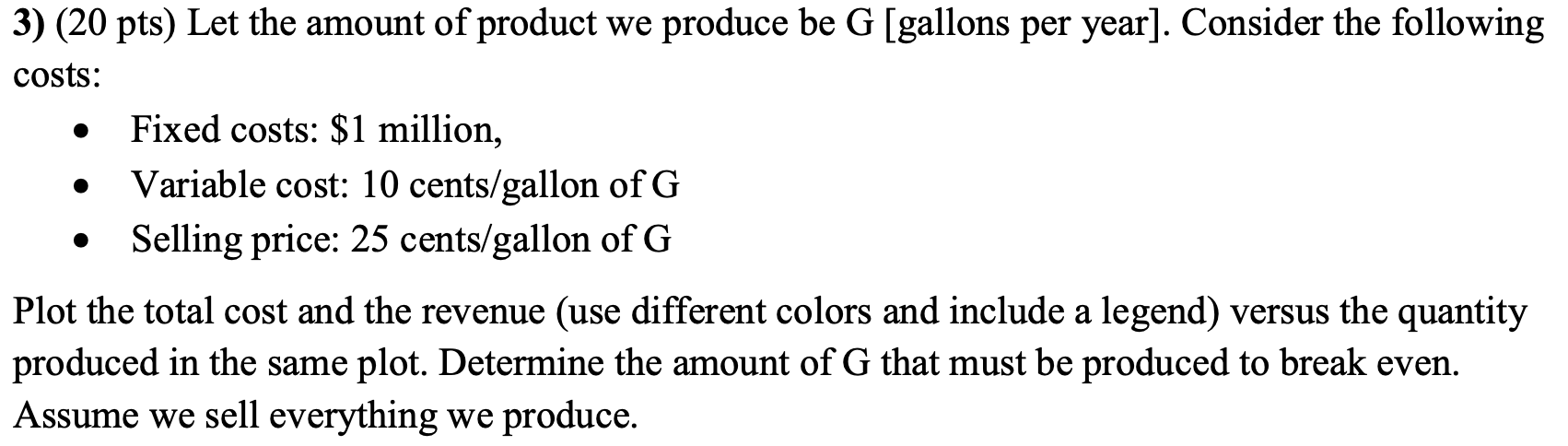# Question Show a step by step on Excel please 3) (20 pts) Let the amount of product we produce be G [gallons per year]. Consider the following costs: Fixed costs: \$1 million, Variable cost: 10 cents/gallon of G Selling price: 25 cents/gallon of G Plot the total cost and the revenue (use different colors and include a legend) versus the quantity produced in the same plot. Determine the amount of G that must be produced to break even. Assume we sell everything we produce.FULGCJ The Asker · Mechanical EngineeringShow a step by step on Excel please

Transcribed Image Text: 3) (20 pts) Let the amount of product we produce be G [gallons per year]. Consider the following costs: Fixed costs: \$1 million, Variable cost: 10 cents/gallon of G Selling price: 25 cents/gallon of G Plot the total cost and the revenue (use different colors and include a legend) versus the quantity produced in the same plot. Determine the amount of G that must be produced to break even. Assume we sell everything we produce.
More
Transcribed Image Text: 3) (20 pts) Let the amount of product we produce be G [gallons per year]. Consider the following costs: Fixed costs: \$1 million, Variable cost: 10 cents/gallon of G Selling price: 25 cents/gallon of G Plot the total cost and the revenue (use different colors and include a legend) versus the quantity produced in the same plot. Determine the amount of G that must be produced to break even. Assume we sell everything we produce.